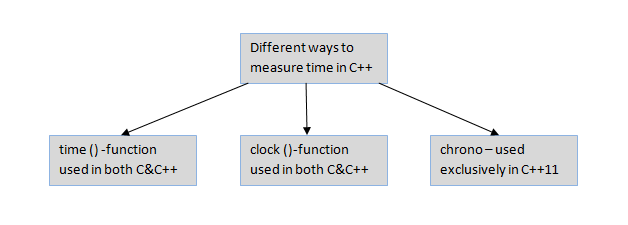×

Search anything:

# Measure time in C++

#### Software Engineering C++Get this book -> Problems on Array: For Interviews and Competitive Programming

Reading time: 25 minutes | Coding time: 10 minutes

You might be wondering how we measure time in C++ and most important is what kind of time we measure in C++? Now, the answer to this question is pretty easy we measure the execution time or CPU time.

Execution time: Execution time or CPU time is the time our machine/pc/CPU takes to complete a task(for example a function). In simple words the total time during which our program is running.

There are multiple ways to measure time in c++ which are listed below:-

1. time()
2. clock()
3. chrono# time() function

time() - returns the current calender time since 1 January 1970 (also Known as Epoch) when argument is NULL or gives the total time elapsed since the start of the function untill it is executed(Total running time of the function).On failure the function returns -1.

• Syntax - time_t time(NULL)
• Output - It gives the value in seconds.
• Parameter - arguments to store time(or result) or NULL pointer.

Let's see the example to understand it easily.

``````#include<iostream>
#include<time>
using namespace std;
int main(){
time_t t = time(NULL);
cout<<"Output:"<<endl;
cout<<t<<endl;
return 0;
}
``````

Output:

``````Output:
1580386755
``````

So it's the time since 1 January 1970 till your current date and time in seconds.
lets see another example.

``````#include<bits/stdc++.h> //Headerfile which include all other headerfiles in c++
using namespace std;
//Function to print fibonacci number
int fib(int n){
if(n==1 or n==0){
return n;
}

return fib(n-1) + fib(n-2);
}
int main(){
time_t start = time(&start);
fib(50);
time_t end = time(&end);
cout<<end-start<<" seconds"endl;
}
``````

Output:

``````193 seconds
``````

Now it's the time taken by my pc to execute the above function.It may vary according to your CPU performance.

# clock() function

clock() - returns the time consumed by the program during processing or number of clock ticks elapsed since an epoch.

• Syntax - clock_t clock(void)
• Output - It gives the output in millisecond but to get the output in second you need to divide the result with CLOCKS_PER_SEC.On error -1 is returned.

Let's see an example.

``````#include<bits/stdc++.h>
using namespace std;

int fib(int n){
if(n==1 or n==0){
return n;
}

return fib(n-1) + fib(n-2);
}
int main(){
clock_t start_time = clock();
fib(50);
clock_t end_time1 = clock();
clock_t result = end_time1 - start_time;
cout<<result<<" milliseconds"<<endl;
cout<<result/CLOCKS_PER_SEC<<" seconds"<<endl;
return 0;
}
``````

Output:

``````192777 milliseconds
192 seconds
``````

# chrono library

Since chrono was introduced in C++11 it has become the stardard way of dealing with time and date in a program because of precision over time considering time and date might be different in different computer so work with a neutral tool chrono library was found which eliminates all the drawbacks that might be found in other methods.
Now the utilities to measure time in chrono library are as follows:

Functions Description
system_clock wall clock time from the system-wide realtime clock
high_resolution_clock the clock with the shortest tick period available
• Syntax - chrono::function_name
• Output - It gives the value in seconds,milliseconds,microseconds based on how we want the output we will discuss with an example.

Program to show chrono library functions

``````#include <iostream>
#include <chrono>
using namespace std;
// main function to measure elapsed time of a C++ program
// using chrono library
int fib(int n){
if(n==1 or n==0){
return n;
}

return fib(n-1) + fib(n-2);
}
int main()
{
fib(40);
cout<<chrono::duration_cast<chrono::nanoseconds>(end-start).count()<<" nanoseconds"<<endl;
cout<<chrono::duration_cast<chrono::microseconds>(end-start).count()<< " microseconds" << endl;
cout<<chrono::duration_cast<chrono::milliseconds>(end-start).count()<<" milliseconds"<<endl;
cout<<chrono::duration_cast<chrono::seconds>(end-start).count()<< " seconds";
return 0;
}
``````

Output

``````1653745600 nanoseconds
1653745 microseconds
1653 milliseconds
1 seconds
``````

The difference between time(),clock() and chrono can easily be seen.

## Question

#### Which one of the following is the best tool to measure time in c++?

chrono
clock()
time()
All of these
Because of the precision over time provided by chrono and removing the possiblities of error involving due difference in time and date and it is fast.

With this article at OpenGenus IQ, you have the basic knowledge of measuring time in C++ and you can use it effectively in production code now. Enjoy.

#### Prateek Sharma

Intern at OpenGenus | Student at Dr. Akhilesh Das Gupta Institute of Technology & Management